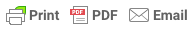Sorry, you do not have permission to ask a question, You must login to ask question. Become VIP Member

The calculation of the load-carrying capacity of under-designed or deteriorated beams is the first step before conducting any repair or rehabilitation work.

The load-carrying capacity evaluation process involves measuring the concrete member’s existing dimensions and estimating its reinforcement area and concrete strength. Additionally, the load acting on the structural element needs to be calculated accurately.

After that, evaluate the beam’s capacity using equations of strength design method and specifications provided by applicable codes like ACI 318-19 and IS 456. Finally, the designer can ascertain the beam’s load-carrying capacity status based on which proper repair method is selected.

In summary, the back-calculation of the beam design process gives the procedure for computing the load-carrying capacity of a reinforced concrete beam.

## How to Calculate Capacity of an existing Beam for Repair Purposes?

1. Measure the span of the slab, which is supported by a beam.
2. Measure the span of the beam.
3. Estimate the live load on the slab based on the function of the building. For instance, use 2.4 KN/m2 (50 psf) for offices, as per Table 4-1 in ASCE standard (ASCE/SEI 10-7).
5. Transfer loads from the slab to the beam. For a one-way slab, half of the total load on the slab goes to a beam from one side, and the other half goes to the other side of the slab. For two way slab, the tributary area can be used to transfer loads to the beams on all sides of the slab.
6. Calculate the load on the reinforced concrete beam. The beam’s dead load is equal to its self-weight and any other dead load from the slab and finishing works. Self-weight is equal to the RC unit weight (24 KN/m3) times the beam’s volume.
7. Compute the ultimate distributed load on the beam using suitable load combinations provided by ACI 318-19.
8. Calculate the ultimate or applied moment on the beam using a suitable equation based on the beam’s support conditions or use finite element modeling.
9. Measure the dimension of the beam, width, and depth.
10. Determine the number and size of embedded steel bars. If the building’s design details are available, the number of bars can be taken from it. However, if the design detail is not available, determine the number of bars using non-destructive tools or break a small portion of the beam to expose steel bars and then count the number of bars.
11. After that, compute the area of reinforcement.
12. Calculate the depth of the rectangular stress block (a). Then, the height of the neutral axis (c).
13. Finally, calculate the design moment of the beam (Md). It should be greater than the applied moment (Mu); otherwise, the beam needs to be rehabilitated.
14. The rehabilitation works rely on the computed design moment and applied moment to redesign the element (adding extra reinforcement or increasing width and depth of the beam or both).

## Example

Compute the capacity of a beam shown in Figure-1. The dimension of the beam is 250 mm width (b), 380 mm height (h), and 350 mm effective depth (d). The thickness of the one-way slab is 100mm. The yield strength of steel bar (fy) is 280 MPa, and concrete compressive strength (fcy’) is 17 MPa.

### Solution:

#### 1. Loads on the RCC Slab

Self-weight= concrete unit weight * Volume of concrete

= 24 * 0.1= 2.4 KN/m2

Live load on Slab= 2.4 KN/m2 (Office use; per Table 4-1 in ASCE standard (ASCE/SEI 10-7)).& Ad Free!

GET VIP MEMBERSHIP NOW!

Finishing loads on slab= 0.8 KN/m2

#### 2. Loads on the Beam

Self-weight= concrete unit weight* beam width*beam height

=24 * 0.28*0.25= 1.68 KN/m

Live load from the slab= 9.6 KN/m

Ultimate distributed load on the beam (Wu)= 1.2*(1.68+12.8)+1.4*9.6= 30.816 KN/m

#### 3. Compute Applied Moment

Assume partial fixity of columns

Applied moment (Mu)= (Wu * l2)/10 = (30.816*5.52)/10=93.218 KN.m

#### 4. Geometry of the Original Section

Width (b) = 250 mm (consider the beam as rectangular section)

Height (h) = 380 mm, and effective depth (d) = 350mm

Used Bars: 4 No. 16

#### 5. Compute Resistant Moment

Reinforcement area (As) = ((PI/4)*D2)* No. of bars = (PI/4)* 162* = 804.24mm2

Depth of rectangular stress block (a) = (As*fy)/ 0.85*fc’*b = (804.24*280)/ 0.85*17*250 = 62.33 mm

Neutral axis (c)= a/0.85= 62.33/0.85= 73.33 mm

c/dt= 73.33/350= 0.209<0.375, hence the strength reduction factor (Phi) is 0.9. c/dt is a value which is used to determine the exact value of strength reduction factors for different concrete elements.

Design Moment (Resistant moment) (Md)= Phi*As*fy(d-0.5a)

Design Moment (Resistant moment)= 0.9*804.24*280(350-(0.5*62.33))= 64617804.82 N.m = 64.61 KN.m

Since, resistance moment= 64.61 KN.m< applied moment= 93.218 KN.m, the beam needs improvement and its load-carrying capacity has to be increased.

## FAQs

How do you calculate the load bearing capacity of a beam?

1. Estimate loads on the beam.
2. Measure the existing dimensions of the concrete member and estimate its reinforcement area and concrete strength.
3. Evaluate the beam’s capacity using equations of strength design method and specifications provided by applicable codes like ACI 318-19 and IS 456.

How to estimate concrete strength of existing concrete beam?

Several test methods are available for the evaluation of the concrete strength of an existing beam, for instance, core cutter test, ultrasonic test, Windsor probe test, etc.

How to calculate self-weight of a beam?

Self-weight is equal to the RC unit weight (24 KN/m^3) times the beam’s volume (cross-section area of the beam times 1 m). The value expressed in weight per unit length.

Design of Rectangular Reinforced Concrete Beam

Non-destructive Tests on RC Structures: Basic Methods, and Purposes

Strengthening Techniques of Reinforced Concrete Beams-Bond Characteristics